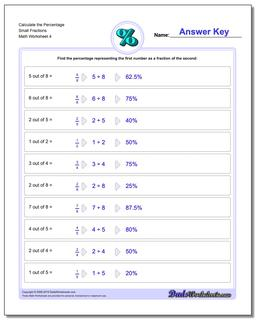# Math Worksheets: Percentages: Percentages: Calculate the Percentage Small Fractions (Fourth Worksheet)## Calculate the Percentage Small Fractions (Fourth Worksheet)

PropertyValue
DescriptionCalculate the Percentage Small Fractions: Percent worksheets where students are given two numbers and must determine what percentage one number is of the other number. (Fourth Worksheet)
Resource TypeWorksheet